Electron. J. Diff. Eqns., Vol. 2004(2004), No. 103, pp. 1-21.

### A nonlinear wave equation with a nonlinear integral equation involving the boundary value Thanh Long Nguyen, Tien Dung Bui

Abstract:
We consider the initial-boundary value problem for the nonlinear wave equation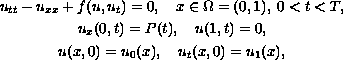where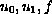are given functions, the unknown function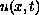and the unknown boundary value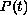satisfy the nonlinear integral equation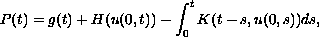where,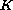,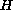are given functions. We prove the existence and uniqueness of weak solutions to this problem, and discuss the stability of the solution with respect to the functions,, and. For the proof, we use the Galerkin method.

Submitted January 14, 2004. Published September 3, 2004.
Math Subject Classifications: 35B30, 35L70, 35Q72.
Key Words: Galerkin method; integrodifferential equations; Schauder fixed point theorem; weak solutions; stability of the solutions

Show me the PDF file (323K), TEX file, and other files for this article.Thanh Long Nguyen Department of Mathematics and Computer Science University of Natural Science Vietnam National University HoChiMinh City 227 Nguyen Van Cu Str., Dist.5, HoChiMinh City, Vietnam email: longnt@hcmc.netnam.vn Tien Dung Bui Department of Mathematics University of Architecture of HoChiMinh City 196 Pasteur Str., Dist. 3, HoChiMinh City, Vietnam